#### Theory of Biomedical Measurements and Transducers

Introduction:

There are numerous physical measurements that are made in medicine. Such can be either invasive or non-invasive. Measurements can be made devoid of physical contact with the body, like in the case of CT or MRI scans.

The other measurements can be made with the help of miniature transducers, like an endoscopy or the measurement of intra-arterial blood pressure. Numerous measurements, though, are carried out in the form of blood tests, where the reaction of blood samples to chemical reagents give information on the condition of an organ or the effectiveness of a bodily function. These tests generally as well include the use of instrumentation to monitor the status of reactions, like the change of colour of a test strip.

In common terms, a transducer is a device that measures a physical phenomenon and transforms the variation in this phenomenon into an electrical signal. This is, hence the signal can then be conditioned and processed with sophistication and precision provided by electronic instrumentation and equipment and then generally stored in electronic or hardcopy form for record and later referral.

Common Physical Measurements:

Most of the physical parameters are generally measured in both invasive and non-invasive settings. Among the most common are the following:

Electricity - Surface Potentials:

These are signals which can be measured from the surface of the body by using electrodes and several examples have been mentioned like the ECG and the EEG is as shown in figure below: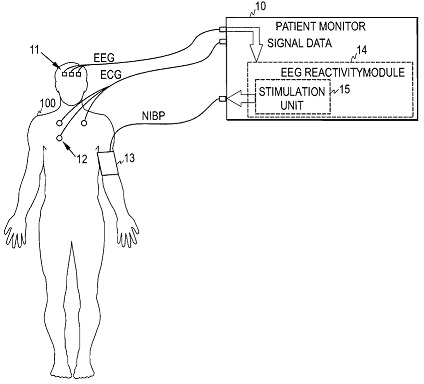Figure: Measurement of EEG and ECG

Pressure:

The pressure can be measured externally as in case of blood pressure measurement, where the gauge pressure in compression cuff is measured as this is deflated to predict the internal pressure in the artery as shown in figure below. Solid-state pressure transducers are accessible for the aim in a diversity of ranges and accuracies. Miniature pressure transducers permit intra-arterial pressure measurement and catheterised insertion of transducers into the heart for long term monitoring. They are as well accessible for other locations like eye.

Temperature:

Since with pressure, the temperature can be measured externally or internally. Sensors can be mounted on the body or inserted at appropriate sites. They generally exploit the property of thermal expansion of metal, or the differing rates of thermal expansion of two distinct metals.

Flow:

The rate of flow of air in and out of the lungs is significant in the diagnosis and treatment of diseases like asthma and lung cancer and also other pulmonary complaints. The flow of blood in narrowed arteries that have suffered stenosis or via damaged valves in the heart is as well a significant measurement that can be accomplished via the use of miniature transducers which can be inserted via catheters.

Strain:

Damage to tendons, muscles and ligaments, as is general in sporting injuries, can be assessed with the help of transducers that can measure the stress and strain that they have been subjected to and can aid in monitoring the healing procedure.

Acceleration:

The technology behind accelerometers has enhanced much in recent years, allowing like transducers to become more compact in size and shape. These can be employed to measure the forces placed on the body in high speed activities like space travel or in general studies of walking gait or limb movement. They have as well been employed more recently to help independent living among the elderly, where they have been employed to monitor falls or unsteadiness in the balance of elderly in their own homes.

Typical Transducer Characteristics:

Most of the solid-state pressure transducers are made in the form of a Wheatstone bridge that is implemented directly on Silicon. The related interfacing and signal conditioning amplifier and any other electronics required can frequently be mounted on similar chip as is employed for the sensor itself. An illustration of the construction of Sensym SPX50 pressure transducer is as shown in figure below. This is fabricated as a piezo-resistive sensor in the form of Wheatstone bridge, comprising of four ion-implanted resistors etched onto an integral silicon diaphragm that transform the shear stress due to applied pressure into the electrical output. The diaphragm is mounted and bonded over the access port where the input pressure is applied. Bridge is exited by using an external supply voltage and can operate over a broad voltage range.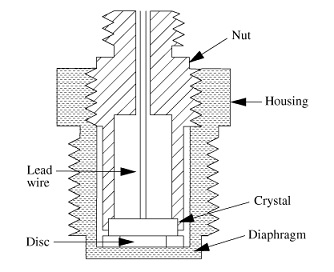Figure: The Physical Construction of a Solid-State Pressure Transducer

The electrical equivalent circuit of transducer is as shown in figure below that can be seen that of an elementary Wheatstone bridge giving a differential output signal. With zero pressure applied to transducer, the potential at both output terminals associative to ground is half the supply voltage. Whenever input pressure is applied to transducer, the potential at the positive output terminal rises from half the supply voltage, whereas that at the negative output terminal reduces. The difference between such two potentials is a small signal voltage that is proportional to the applied pressure.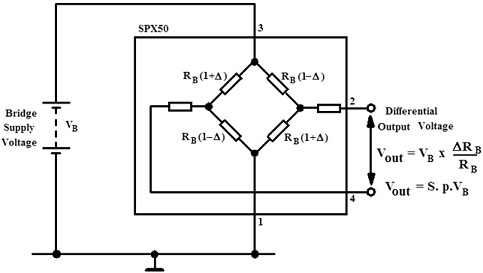Figure: Equivalent Circuit of the SPX 50 Pressure Transducer

The performance characteristics of transducer are given in table shown below. This table exhibits the parameters that explain how the transducer operates and ranges that apply to the values of such parameters, due to manufacturing variations and the ambient conditions in which the transducer is employed.

Table: The Performance Parameters of SX 50 Pressure Transducer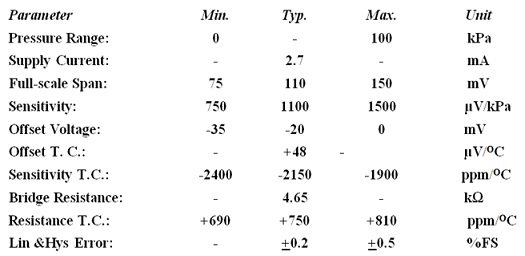Figure exhibits a plot of transducer transfer characteristic, from which it can be observe, that there considerable variation in the transducer properties due to the manufacturing variation.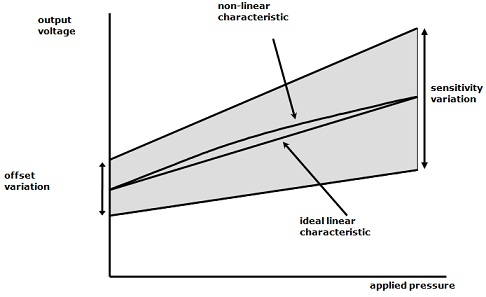Figure: Transfer Characteristic of SX 50 Pressure Transducer

The output voltage at positive output terminal, pin 2, of the transducer is as follows:

V+ = [{RB(1 + Δ)} / {RB(1 - Δ) + RB(1 + Δ)}] VB = [{RB(1 + Δ)}/2RB] VB

Where ΔRB is the change in resistance RB, of an arm of bridge that is proportional to the applied pressure and VB is the bridge supply voltage.

The output voltage at negative output terminal, pin 4, of the transducer is as follows:

V- = [{RB(1 - Δ)} / {RB(1 + Δ) + RB(1 - Δ)}] VB = [{RB(1 - Δ)}/2RB] VB

The differential output of sensor is then as follows: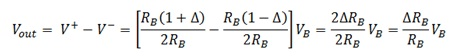If a span sensitivity S, is specified for transducer as the output voltage in mV per unit of supply voltage VB, per unit of the applied pressure p that is as mVV-1kPa-1 then the ideal transducer output can be stated as:

Vout = V+ - V- = SVBP

In practice, there are very large deviations from the ideal in transducer characteristic, as can be observe in figure shown above. The bridge output is not zero for zero applied pressure, however an offset voltage, VOS, exists that has an associated range due to the manufacturing variations. That is to state, whenever zero input pressure is applied, the two output terminals of transducer do not sit at a potential of VB/2, that is, half of the bridge supply voltage, however both can differ slightly from this.

This signifies that the differential output voltage measured among the two terminals might be some negative or positive value that is not zero. This changes the output voltage to:

Vout = V+ - V- = VOS + SVBP

Where the offset voltage VOS, is very small in relation to bridge supply voltage however can be quite large whenever considered in relation to differential output voltage related with the pressure measurement range.

Additionally, most of the transducers encompass a large manufacturing variation in the sensitivity of diaphragm and as a result there is a large variation in the span sensitivity from one transducer to the subsequent. This signifies that the parameter S has a large variation and hence the full-scale output voltage from transducer for a given bridge supply voltage differs considerably. In addition, both the offset voltage and span sensitivity differ with temperature and as a result encompass associated temperature coefficients. This signifies that if nominal values of such parameters are quoted at a given temperature, T0, they will differ as the temperature deviates from this value, and hence they should be treated effectively as functions of temperature. In this case, the transducer output is as follows:

Vout = V+ - V- = VOSO[1 + α(T -To)] + So[1 + β(T -To)] VBP

Where,

T0 = reference temperature at which the nominal parameter values are quoted
T = actual operating temperature
VB = bridge supply voltage
p = applied input pressure
VOS0 = nominal value of offset voltage
S0 = nominal value of the span sensitivity
α = temperature coefficient of offset voltage
β = temperature coefficient of span sensitivity

To further complicate the matters, transducer characteristic has certain degree of non-linearity over its range of operation and deviates from ideal straight-line output voltage vs. pressure relationship as shown in figure above. Finally, properties like hysteresis and ageing effects can as well give rise to the measurement errors.

Transducer Calibration and the Compensation:

Such sources of error can only be counteracted by passing the output of transducer via a signal conditioning amplifier as shown in figure below: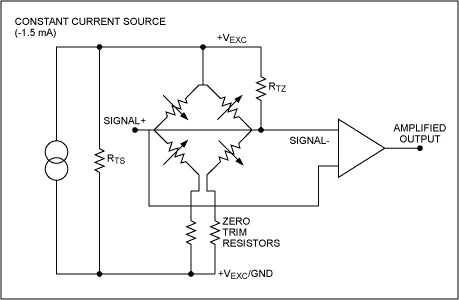Figure: Signal Conditioning of a Silicon Pressure Transducer

The offset voltage is cancelled by adding an equivalent and opposite cancellation voltage, VOC, in conditioning amplifier. It is completed in the first instance at reference temperature, T0. Variation in span sensitivity is counteracted by adjusting the profit of amplifier at the reference temperature to provide the necessary maximum output voltage for full-scale input pressure. The bridge supply voltage can as well be employed for this purpose.

The temperature coefficient of offset voltage is compensated for by making sure that the equivalent and opposite offset cancellation voltage consists of a temperature coefficient of similar magnitude as that of the offset voltage. The bridge supply voltage can as well be made to differ with temperature and should have a temperature coefficient that is equivalent and opposite to that of span sensitivity. Whenever this is implemented, the output voltage from the conditioning amplifier is as follows:

Vout = Voso (1 + α ΔT) + Voco (1 + γ ΔT) + GSo (1 + β ΔT) VBo (1 + δ ΔT)p

Where:

ΔT = T - T0 is the deviation in temperature from nominal reference value
γ = temperature coefficient of offset cancellation voltage
δ = temperature coefficient of the bridge supply voltage

In order to get full cancellation and calibrate the output voltage to be independent of temperature needs:

Voso (1 + α ΔT) + Voco (1 + γ ΔT) + GSo (1 + β ΔT) VBo (1 + δ ΔT)p = G SoVBO p

This needs:

Voso (1 + α ΔT) + Voco (1 + γ ΔT) + GSo VBO (1 + β ΔT + δ ΔT + β δ ΔT2)p = G SoVBO p

When the second order term in ΔT2 is avoided as negligible then full compensation needs:

VOCO = - VOSO; γ = α; δ = -β

More precisely if the first two conditions are satisfied we require:

β ΔT + δ ΔT + β δ ΔT2 = 0

β ΔT + δ(1 + β ΔT) ΔT = 0

As ΔT can’t be taken as zero this needs:

β = - δ (1 + β ΔT)

And hence,

δ = - β/(1 + β ΔT) → - β

Such values are only computed for design purposes and perfect matching for compensation is attained throughout the calibration procedure. A simple calibration process is as follows:

• Set the ambient temperature to lower value (that is, this might be the reference temperature T0 however doesn’t have to be).
• Set input applied pressure to zero.
• Adjust the nominal value of offset cancellation voltage, VOC0, to obtain an output voltage of zero from conditioning amplifier.
• Now set the applied pressure to its full scale value.
• Adjust the gain G, or nominal bridge supply voltage VB0, to fetch the yield of the conditioning amplifier to specified full-scale value.
• Increase the ambient temperature to its higher value.
• Put the applied input pressure to 0 (zero).
• Adjust the temperature coefficient of offset cancellation voltage to get zero output voltage from conditioning amplifier.
• Increase the applied input pressure to its full-scale value.
• Adjust the temperature coefficient of bridge supply voltage to bring the output voltage of conditioning amplifier to its full-scale value.

Latest technology based Electrical Engineering Online Tutoring Assistance

Tutors, at the www.tutorsglobe.com, take pledge to provide full satisfaction and assurance in Electrical Engineering help via online tutoring. Students are getting 100% satisfaction by online tutors across the globe. Here you can get homework help for Electrical Engineering, project ideas and tutorials. We provide email based Electrical Engineering help. You can join us to ask queries 24x7 with live, experienced and qualified online tutors specialized in Electrical Engineering. Through Online Tutoring, you would be able to complete your homework or assignments at your home. Tutors at the TutorsGlobe are committed to provide the best quality online tutoring assistance for Electrical Engineering Homework help and assignment help services. They use their experience, as they have solved thousands of the Electrical Engineering assignments, which may help you to solve your complex issues of Electrical Engineering. TutorsGlobe assure for the best quality compliance to your homework. Compromise with quality is not in our dictionary. If we feel that we are not able to provide the homework help as per the deadline or given instruction by the student, we refund the money of the student without any delay.